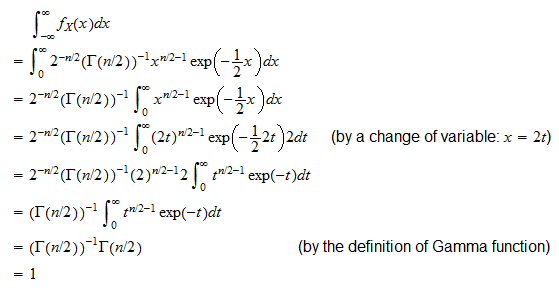StatLect

# Legitimate probability density functions

This lecture discusses two properties characterizing probability density functions (pdfs).

Not only any pdf satisfies these two properties, but also any function that satisfies them is a legitimate pdf.

Therefore, in order to determine whether a function is a valid pdf, we just need to verify that the two properties hold.## Properties of probability density functions

The following proposition formally describes the two properties.

Proposition Letbe a continuous random variable. Its probability density function, denoted by, satisfies the following two properties:

1. Non-negativity:for any;

2. Integral overequals:.

Proof

Remember that, by the definition of a pdf,is such thatfor any interval. Probabilities cannot be negative, thereforeandfor any interval. But the above integral can be non-negative for all intervalsonly if the integrand function itself is non-negative, that is, iffor all. This proves property 1 above (non-negativity).

Furthermore, the probability of a sure thing must be equal to. Sinceis a sure thing, then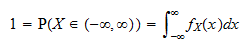which proves property 2 above (integral overequals).

## How to check that a pdf is valid

Any pdf must satisfy property 1 and 2 above. It can be demonstrated that also the converse holds: any function enjoying these properties is a pdf.

Proposition Letbe a function satisfying the following two properties:

1. Non-negativity:for any;

2. Integral overequals:.

Then, there exists a continuous random variablewhose pdf is.

The practical implication is that we only need to verify that these two properties hold when we want to prove that a function is a valid pdf.

## How to build valid pdfs

The proposition above also gives us a powerful method for constructing probability density functions.

Take any non-negative function(non-negative means thatfor any).

If the integralexists and is finite and strictly positive, then defineSinceis strictly positive,is non-negative and it satisfies Property 1.

The functionalso satisfies Property 2 because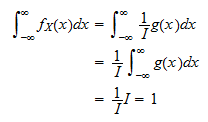Thus, any non-negative functioncan be used to build a pdf if its integral overexists and is finite and strictly positive.

Example Define a functionas follows:How do we construct a pdf from? First, we need to verify thatis non-negative. But this is true becauseis always non-negative. Then, we need to check that the integral ofoverexists and is finite and strictly positive:Having verified thatexists and is finite and strictly positive, we can define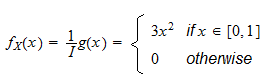By the above proposition,is a legitimate pdf.

## Solved exercises

Below you can find some exercises with explained solutions.

### Exercise 1

Consider the following function: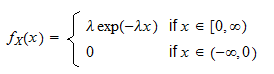where.

Prove thatis a legitimate probability density function.

Solution

Sinceand the exponential function is strictly positive,for any, so the non-negativity property is satisfied. The integral property is also satisfied because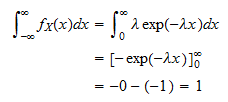### Exercise 2

Define the functionwhereand.

Prove thatis a valid probability density function.

Solutionimplies, sofor anyand the non-negativity property is satisfied. The integral property is also satisfied because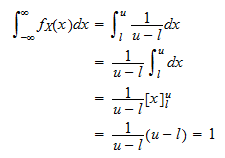### Exercise 3

Consider the function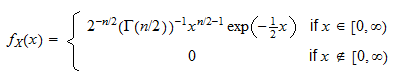whereandis the Gamma function.

Determine whetheris a valid probability density function.

Solution

Remember the definition of Gamma function:is obviously strictly positive for any, sinceis strictly positive andis strictly positive on the interval of integration (except atwhere it is). Therefore,satisfies the non-negativity property because the four factors in the product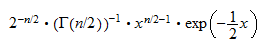are all non-negative on the interval.

The integral property is also satisfied because